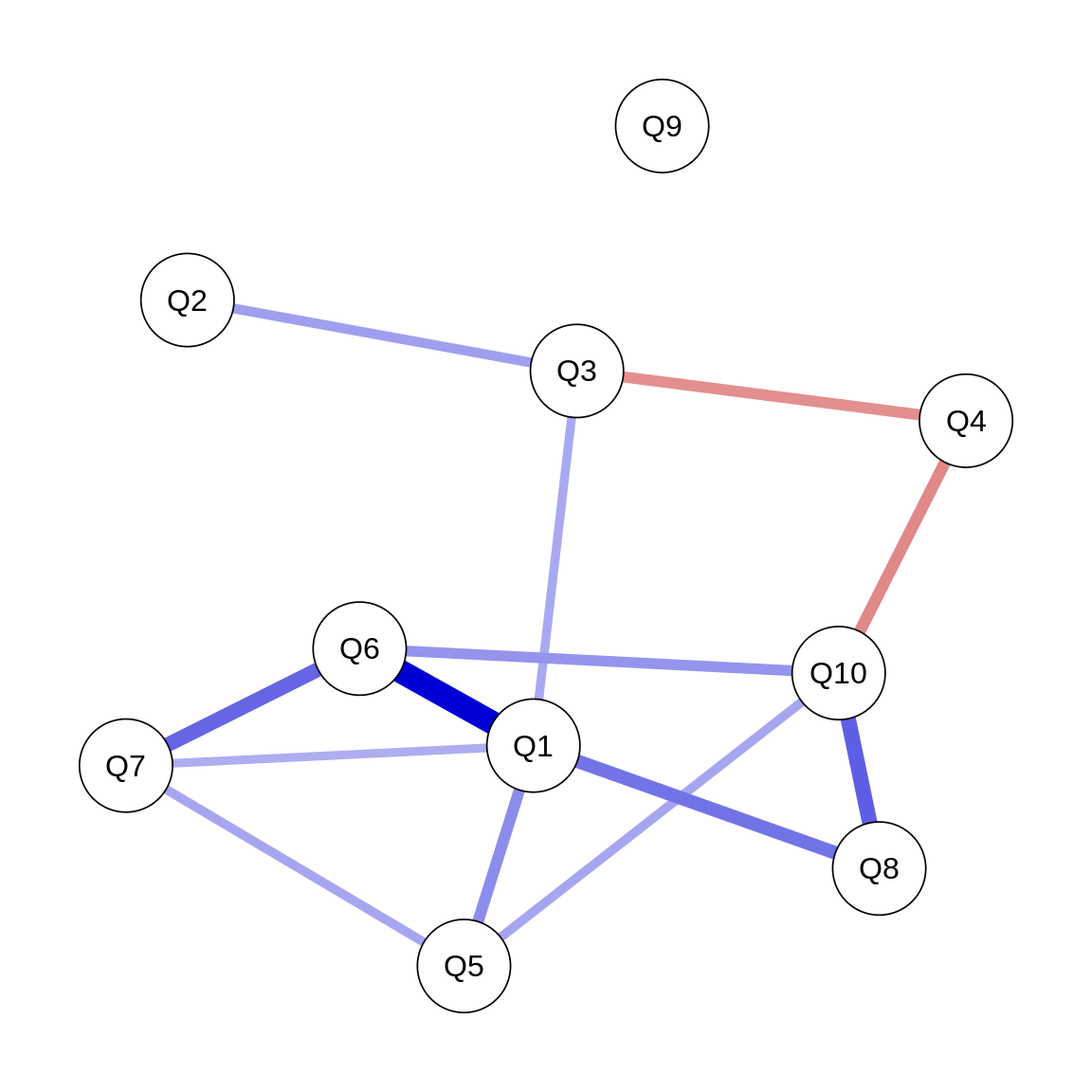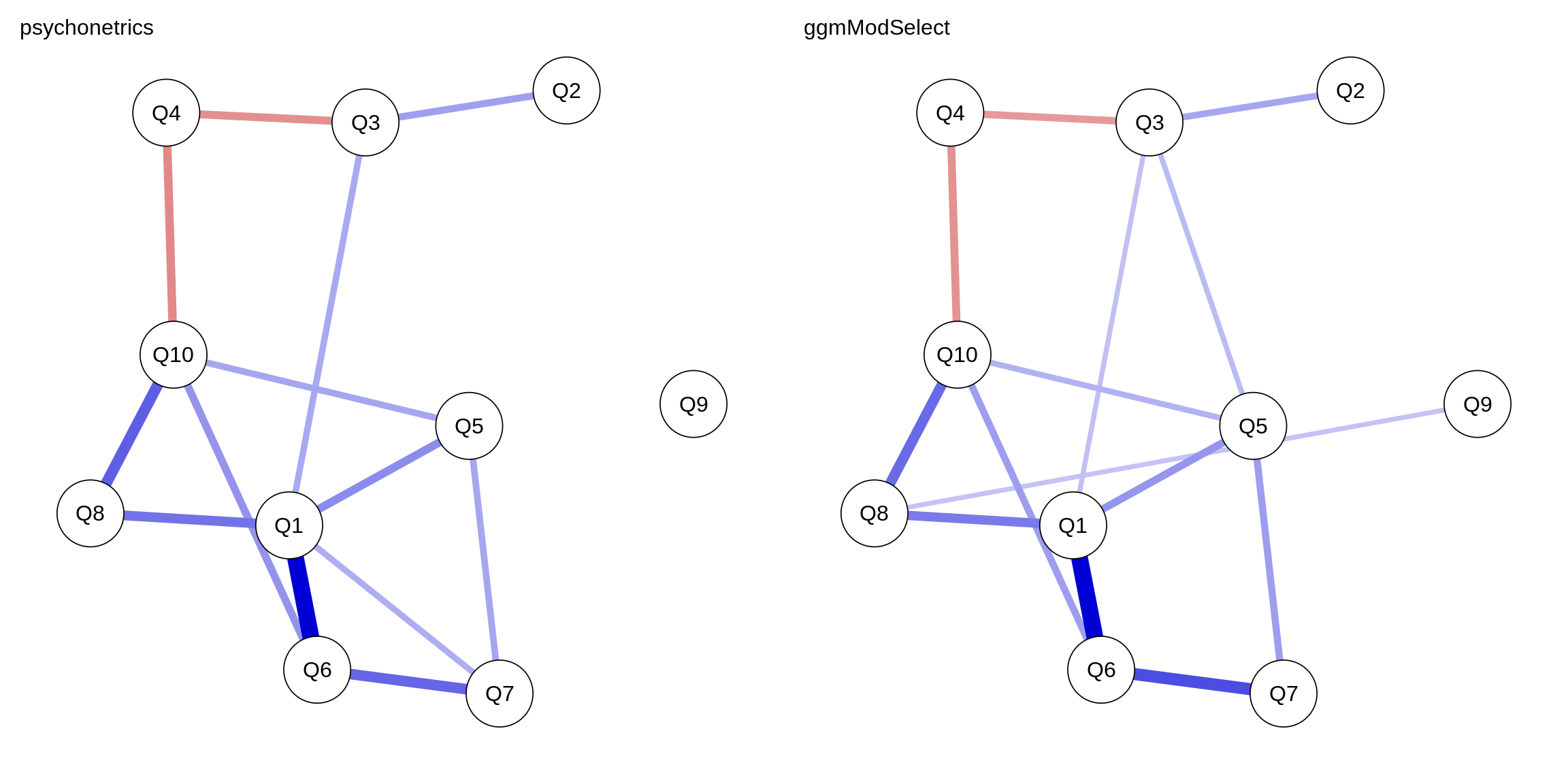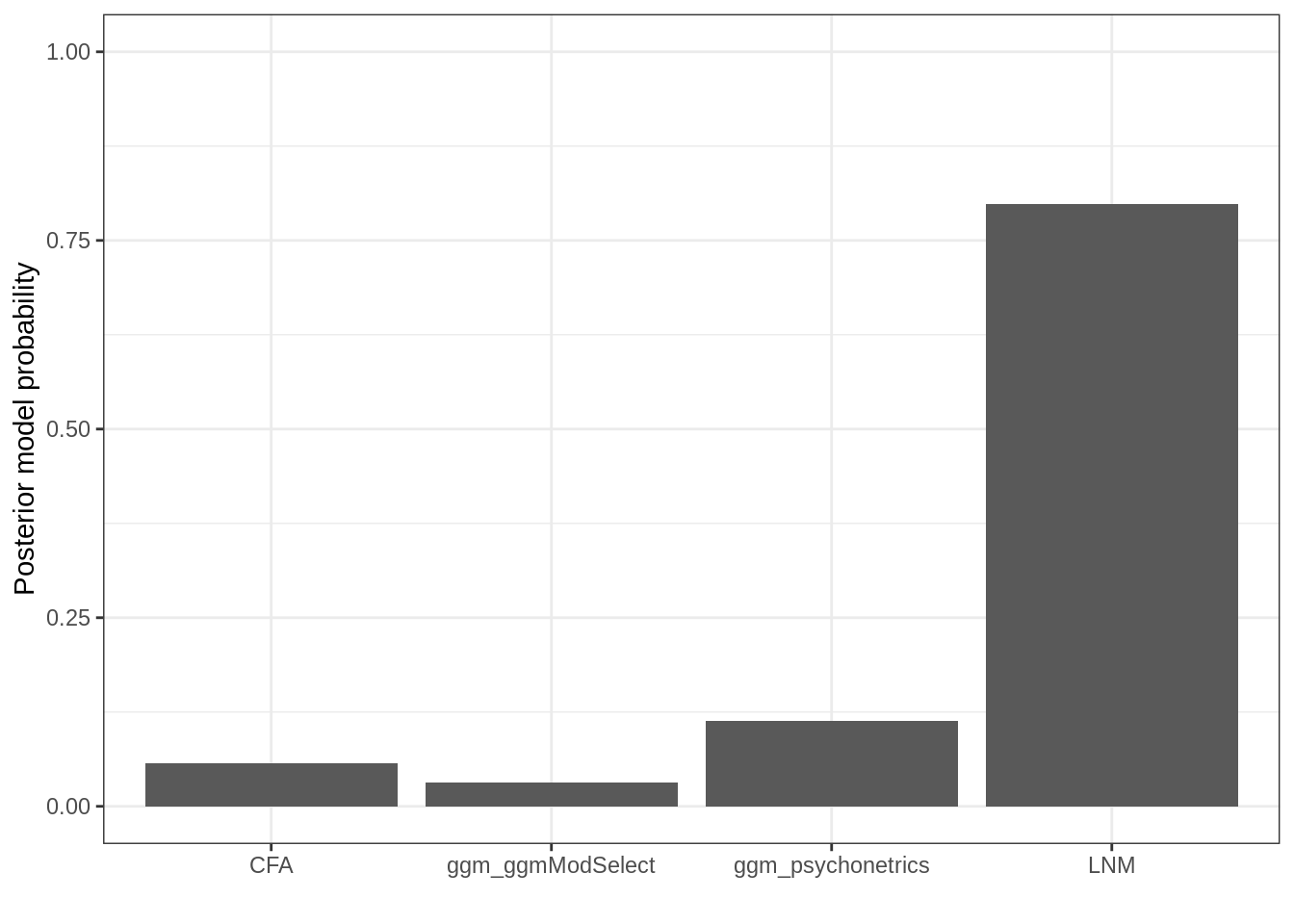# Load all packages:
library("psychonetrics")
library("dplyr")
library("qgraph")
library("ggplot2")
library("bootnet")
library("Rmpfr")
library("semPlot")

# Star Wars Analysis

## Data Preperation

First we can load the data and make a vector of the observed variables:

# Load the data:
data("StarWars")

# Observed variables:
obsvars <- c("Q1", "Q2", "Q3", "Q4", "Q5", "Q6", "Q7", "Q8", "Q9", "Q10")

## GGM Analysis

Next, we can form our first model: a GGM model with all edges included:

# Form GGM model:
ggm1 <- ggm(StarWars, vars = obsvars)

# Run model:
ggm1 <- ggm1 %>% runmodel
## Estimating baseline model...
## Estimating model...
## Computing Fisher information...
## Computing modification indices...

If we are interested in the parameter values and significance of edges, we can use the following command:

# Check parameters:
ggm1 %>% parameters

Next, we can automatically prune all edges:

# Prune non-significant:
ggm1 <- ggm1 %>%
prune(adjust = "fdr", alpha = 0.01)

We can check the modification indices to see wich edges we can add now to improve fit:

# Check modification indices:
ggm1 %>% MIs
##
## Top 10 modification indices:
##
##  var1 op var2      est mi pmi epc matrix row col      group group_id
##    Q1 ~1 <NA> 2.760148                mu   1   1 fullsample        1
##    Q2 ~1 <NA> 4.188192                mu   2   1 fullsample        1
##    Q3 ~1 <NA> 2.819188                mu   3   1 fullsample        1
##    Q4 ~1 <NA> 3.214022                mu   4   1 fullsample        1
##    Q5 ~1 <NA> 2.435424                mu   5   1 fullsample        1
##    Q6 ~1 <NA> 2.686347                mu   6   1 fullsample        1
##    Q7 ~1 <NA> 2.996310                mu   7   1 fullsample        1
##    Q8 ~1 <NA> 2.383764                mu   8   1 fullsample        1
##    Q9 ~1 <NA> 3.247232                mu   9   1 fullsample        1
##   Q10 ~1 <NA> 2.118081                mu  10   1 fullsample        1

To automatically add edges at $$\alpha = 0.05$$ until BIC can no longer be improved, use the following function:

# Stepup estimation:
ggm1 <- ggm1 %>% stepup(criterion = "bic", alpha = 0.05)

This process can be simplified with a single Dplyr pipe!

### Slide 33 ###
# Form GGM model:
ggm1 <- ggm(StarWars, vars = obsvars)

# Run model and estimate structure:
ggm1 <- ggm1 %>% runmodel %>%
prune(adjust = "fdr", alpha = 0.01) %>%
stepup(criterion = "bic", alpha = 0.05)

We can look at fit indices (although they will likely be good):

# Model fit:
ggm1 %>% fit
##            Measure    Value
##               logl -4131.26
##  unrestricted.logl -4109.06
##      baseline.logl -4322.24
##               nvar       10
##               nobs       65
##               npar       33
##                 df       32
##          objective    12.11
##              chisq    44.39
##             pvalue    0.071
##     baseline.chisq   426.35
##        baseline.df       45
##    baseline.pvalue       ~0
##                nfi     0.90
##               pnfi     0.64
##                tli     0.95
##               nnfi     0.95
##                rfi     0.85
##                ifi     0.97
##                rni     0.97
##                cfi     0.97
##              rmsea    0.038
##     rmsea.ci.lower       ~0
##     rmsea.ci.upper    0.063
##       rmsea.pvalue     0.77
##             aic.ll  8328.52
##            aic.ll2  8337.98
##              aic.x   -19.61
##             aic.x2   110.39
##                bic  8447.39
##               bic2  8342.75
##            ebic.25  8523.37
##             ebic.5  8599.36
##            ebic.75  8660.14
##              ebic1  8751.33

Now, we can extract the network and plot it:

# Obtain network:
net1 <- getmatrix(ggm1, "omega")

# Plot:
qgraph(net1,
layout = "spring",
theme = "colorblind",
labels = obsvars)## Network from bootnet

We can also use bootnet for the model estimation:

# Estimate model using bootnet:
mod <- estimateNetwork(StarWars[,obsvars], default = "ggmModSelect")
structure <- 1*(mod$graph!=0) # Form second GGM model with fixed structure: ggm2 <- ggm(StarWars, omega = structure, vars = obsvars) # ERun the model: ggm2 <- ggm2 %>% runmodel # Obtain network: net2 <- getmatrix(ggm2, "omega") This will likely be slightly different from the network estimated by psychonetrics. We can plot both networks next to each other: # Obtain average layout (function from qgraph) Layout <- averageLayout(net1,net2) # plot both networks: layout(t(1:2)) qgraph(net1, layout = Layout, theme = "colorblind", title = "psychonetrics", labels = obsvars) qgraph(net2, layout = Layout, theme = "colorblind", title = "ggmModSelect", labels = obsvars)We can even statistically compare these networks: ### Slide 36 ### compare(psychonetrics = ggm1, ggmModSelect = ggm2) ## model DF AIC BIC RMSEA Chisq Chisq_diff DF_diff ## 1 ggmModSelect 31 8327.476 8449.948 0.03510471 41.35289 NA NA ## 2 psychonetrics 32 8328.515 8447.385 0.03780140 44.39182 3.038923 1 ## p_value ## 1 NA ## 2 0.08128984 ## Confirmatory Factor Analysis To perform a confirmatory factor analysis, we first need to specify the factor structure: # Factor loadings matrix: Lambda <- matrix(0, 10, 3) Lambda[1:4,1] <- 1 Lambda[c(1,5:7),2] <- 1 Lambda[c(1,8:10),3] <- 1 print(Lambda) ## [,1] [,2] [,3] ## [1,] 1 1 1 ## [2,] 1 0 0 ## [3,] 1 0 0 ## [4,] 1 0 0 ## [5,] 0 1 0 ## [6,] 0 1 0 ## [7,] 0 1 0 ## [8,] 0 0 1 ## [9,] 0 0 1 ## [10,] 0 0 1 Next, we can make a vector with names for the latent variables: # Latents: latents <- c("Prequels","Original","Sequels") Now, we can form the CFA model and run it. We use the lvm model function (latent variable model) for this: # Make CFA model: cfamod <- lvm(StarWars, lambda = Lambda, vars = obsvars, identification = "variance", latents = latents) # Run model: cfamod <- cfamod %>% runmodel ## Estimating baseline model... ## Estimating model... ## Computing Fisher information... ## Computing modification indices... We can look at some fit indices of this model: cfamod@fitmeasures[c("chisq","pvalue","rmsea","cfi")] ##$chisq
##  34.57911
##
## $pvalue ##  0.2582765 ## ##$rmsea
##  0.02373261
##
## $cfi ##  0.9879924 These perfectly reproduce the famous lavaan package! library("lavaan") ## This is lavaan 0.6-6.1487 ## lavaan is BETA software! Please report any bugs. ## ## Attaching package: 'lavaan' ## The following object is masked from 'package:psychonetrics': ## ## duplicationMatrix lavmod <- ' Prequels =~ Q1 + Q2 + Q3 + Q4 Original =~ Q1 + Q5 + Q6 + Q7 Sequels =~ Q1 + Q8 + Q9 + Q10 ' lavfit <- cfa(lavmod,StarWars,std.lv=TRUE) fitMeasures(lavfit)[c("chisq","pvalue","rmsea","cfi")] ## chisq pvalue rmsea cfi ## 34.57911327 0.25827655 0.02373261 0.98799236 ## Latent Network Model Next, we can form an LNM model in the exact same way, using the lnm function (alternatively, we can use lvm(..., latent = "ggm")): # Latent network model: lnmmod <- lnm(StarWars, lambda = Lambda, vars = obsvars, identification = "variance", latents = latents) We can run this model and estimate a latent network structure with the same algorithm we used before: # Run model and estimate latent structure: lnmmod <- lnmmod %>% runmodel %>% prune(adjust = "fdr", alpha = 0.01) %>% stepup(criterion = "bic", alpha = 0.05) Finally, we can compare all models: # Compare all models: comparison <- compare( ggm_psychonetrics = ggm1, ggm_ggmModSelect = ggm2, CFA = cfamod, LNM = lnmmod) print(comparison) ## model DF AIC BIC RMSEA Chisq Chisq_diff DF_diff ## 1 CFA 30 8322.703 8448.777 0.02373261 34.57911 NA NA ## 2 ggm_ggmModSelect 31 8327.476 8449.948 0.03510471 41.35289 6.773781 1 ## 3 LNM 31 8321.016 8443.488 0.02152480 34.89233 6.460568 0 ## 4 ggm_psychonetrics 32 8328.515 8447.385 0.03780140 44.39182 9.499491 1 ## p_value ## 1 NA ## 2 0.009250668 ## 3 0.000000000 ## 4 0.002055289 The BICs are close, so one thing we could do is transform these to posterior model probabilities: # To posterior model probability: BICs <- mpfr(comparison$BIC, 100)
BICtrans <- exp(-0.5 * BICs)
sumBICtrans <- sum(BICtrans)
comparison$modelProbability <- as.numeric(BICtrans / sumBICtrans) # Make a plot: g <- ggplot(comparison, aes(x=model, y = modelProbability)) + geom_bar(stat="identity") + xlab("") + ylab("Posterior model probability") + theme_bw() + ylim(0,1) print(g)The latent network model performs best in this case! Finally, we can make a path diagram. This is not very trivial… but can be done with the semPlot package: # LISREL Model: LY = Lambda (lambda-y), TE = Theta (theta-epsilon), PS = Psi mod_lisrel <- lisrelModel(LY = getmatrix(lnmmod, "lambda"), PS = getmatrix(lnmmod, "sigma_zeta"), TE = getmatrix(lnmmod, "sigma_epsilon"), latNamesEndo = latents, manNamesEndo = obsvars) # Change latent to pcors: omega <- getmatrix(lnmmod, "omega_zeta") mod_lisrel@Pars$std[23:28] <- mod_lisrel@Pars$est[23:28] <- omega[lower.tri(omega,diag=TRUE)] # We can make this nicer (set whatLabels = "none" to hide labels): graph <- semPaths(mod_lisrel, what = "std", # this argument controls what the color of edges represent. In this case, standardized parameters whatLabels = "omit", # This argument controls what the edge labels represent. In this case, parameter estimates # as.expression = c("nodes","edges"), # This argument draws the node and edge labels as mathematical exprssions style = "lisrel", # This will plot residuals as arrows, closer to what we use in class residScale = 10, # This makes the residuals larger theme = "colorblind", # qgraph colorblind friendly theme layout = "tree2", # tree layout options are "tree", "tree2", and "tree3" cardinal = "lat cov", # This makes the latent covariances connet at a cardinal center point curvePivot = TRUE, # Changes curve into rounded straight lines sizeMan = 4, # Size of manifest variables sizeLat = 10, # Size of latent varibales edge.label.cex = 1, mar = c(6,1,8,1), # Sets the margins reorder = FALSE, # Prevents re-ordering of ovbserved variables # filetype = "pdf", # Store to PDF # filename = "semPlotExample1", # Set the name of the file width = 8, # Width of the plot height = 5, # Height of plot groups = "latents", # Colors according to latents, pastel = TRUE, # Pastel colors borders = FALSE, nCharNodes = 0, # Disable borders DoNotPlot = TRUE # Prevents plotting for now ) graph$Edgelist\$directed[23:26] <- FALSE # We need to make the latent structure undirected not bidirectional
plot(graph) # Plot the results